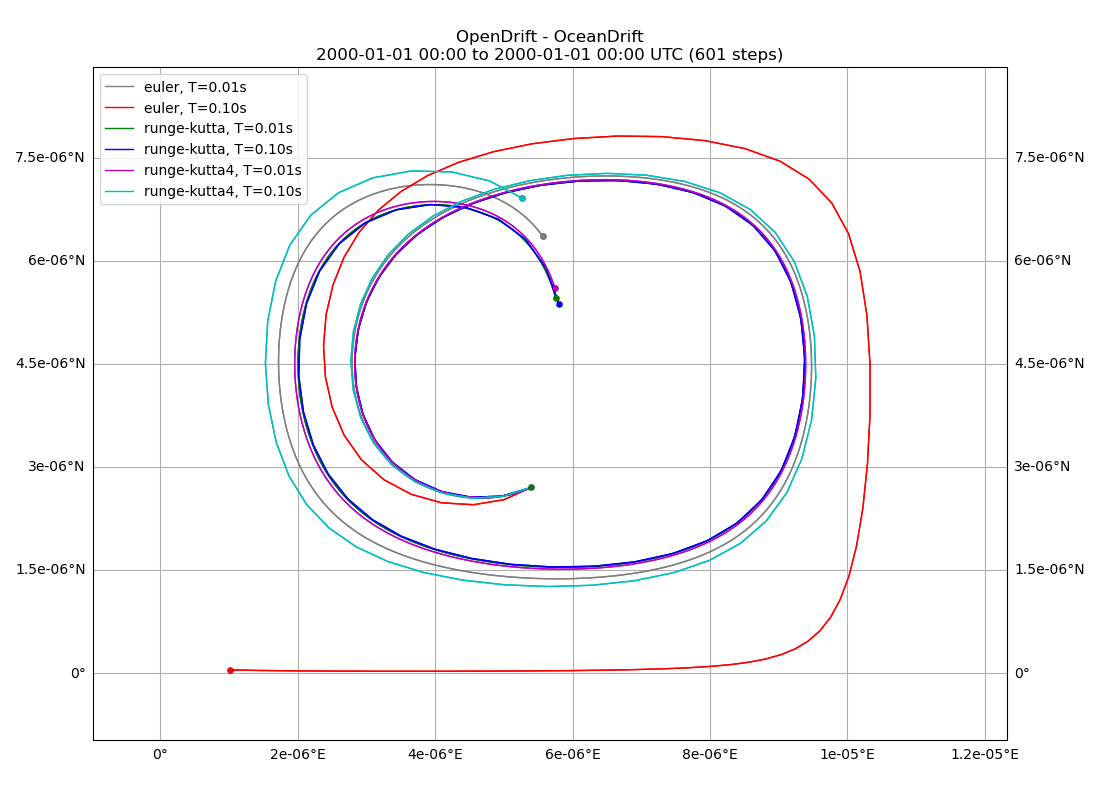Illustrating the difference between Euler and Runge-Kutta propagation schemes, using an idealised (analytical) eddy current field.```euler, T=0.01s
euler, T=0.10s
runge-kutta, T=0.01s
runge-kutta, T=0.10s
runge-kutta4, T=0.01s
runge-kutta4, T=0.10s
1.0 ALPHA

(<GeoAxes: title={'center': 'OpenDrift - OceanDrift\n2000-01-01 00:00 to 2000-01-01 00:00 UTC (601 steps)'}>, <Figure size 1100x808.707 with 1 Axes>)
```

```import numpy as np
from datetime import datetime, timedelta

from opendrift.models.oceandrift import OceanDrift

duration=timedelta(seconds=6)
x = [.6]
y = [.3]
lon, lat = double_gyre.xy2lonlat(x, y)

runs = []
leg = []
i = 0
for scheme in ['euler', 'runge-kutta', 'runge-kutta4']:
for time_step  in [0.01, 0.1]:
leg.append(scheme + ', T=%.2fs' % time_step)
print(leg[-1])
o = OceanDrift(loglevel=50)